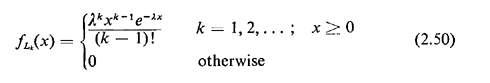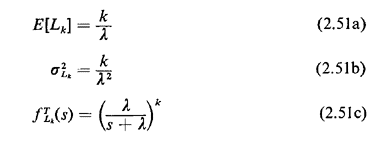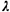## 2.11.3 Erlang PDF

A random variable Lk is said to be a kth-order Erlang random variable if its pdf is given byThe mean, variance, and s-transforms areThe Erlang pdf arises frequently in the application of Poisson processes. As we will show in Section 2.12, the kth-order Erlang pdf describes the probabilistic behavior of time until the kth arrival in a Poisson process. Note that for k = 1, the Erlang reduces to the familiar negative exponential pdf.

In fact, Lk may be thought of as the sum of k independent, identically distributed negative exponential random variables, each with mean 1/; this provides a convenient way to understand intuitively the Erlang pdf and to remember the mean, variance, and s-transform.